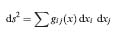تخطي للمحتوى الرئيسي تخطي لصندوق البحث

Definition: elliptic geometry from The Hutchinson Dictionary of Scientific Biography

Subject: maths and statistics

System of non-Euclidean geometry developed as the initial form of Riemann geometry, and regarding all geometrical operations as carried out in ‘curved’ space, for example, as though on the surface of an ellipsoid or sphere. A ‘straight line’ is thus defined (then) as the shortest curve (geodesic) on the curved surface joining two points.

Summary Article: Riemannian geometry
From The Penguin Dictionary of Mathematics

A type of non-Euclidean geometry developed by Riemann in 1854. In Euclidean geometry, the distance between two neighbouring points on a plane is given by a relationship of the form

ds2 = dx2 + dy2

where rectangular Cartesian coordinates are used. More generally, the relationship can be written as

ds2 = Adx2 + B dx dy + C dy2

where A, B, and C depend on x and y.Gauss considered this case and showed that it is possible to determine the curvature at a point intrinsically in terms of A, B, and C. Riemann generalized this approach into the study of any type of metric space in any number of dimensions. What is now called a Riemannian space is a space with n coordinates (x1, x2, …, xn) in which the distance between neighbouring points is given by a quadratic form,where the gij(x) are functions of x1, x2, …, xn. In the original form of Riemannian geometry, ds2 was required to be always positive, although this is not the case in applications to general relativity theory. Usually, the coefficients gij(x) are taken to have a nonvanishing determinant. The gij(x) are the components of a symmetric covariant tensor field (the metric tensor).In Riemannian geometry, the distance between two points can be determined by an integral of ds. Riemannian curvature is defined by an expression involving the metric tensor of the Riemannian space and a tensor known as the Riemann-Christoffel curvature tensor after Gauss and Elwin Bruno Christoffel (1829-1900).

Riemannian geometry had a profound effect on the way people thought about geometry and on the development of tensor analysis. It was also essential in the formulation of general relativity and in later attempts to develop a unified field theory. The term is sometimes used in a more restricted sense to describe a particular type of non-Euclidean geometry in which the plane is interpreted as a sphere and a line as a great circle on the sphere. In this form of non-Euclidean geometry, Euclid's parallel postulate is replaced by the postulate that no line can be drawn parallel to a given line through a point lying outside the line. Moreover, Euclid's second postulate (that a line can be extended indefinitely in both directions) is not applicable. This non-Euclidean geometry is also called elliptic geometry.Copyright © Penguin Books Ltd, 1989, 1998, 2003, 2008

مقالات كريدو ذات الصلة

Full text Article Riemannian geometry
The Penguin Dictionary of Mathematics

A type of non-Euclidean geometry developed by Riemann in 1854. In Euclidean geometry, the distance between two neighbouring points on a plane...

Full text Article Non-Euclidean geometries
Philosophy of Science A-Z

Alternatives to Euclidean geometry developed as rigorous geometrical systems in the nineteenth century. They deny Euclid’s fifth postulate. ...

Full text Article Riemannian geometry
Academic Press Dictionary of Science and Technology

1. The geometry of Riemannian manifolds; i.e., those having an infinitesimal squared distance ds that is everywhere positive. 2. ...

مشاهدة المزيد من كريدو